×#### Thank you for registering.

One of our academic counsellors will contact you within 1 working day.

Click to Chat

1800-1023-196

+91-120-4616500

CART 0

• 0

MY CART (5)

Use Coupon: CART20 and get 20% off on all online Study Material

ITEM
DETAILS
MRP
DISCOUNT
FINAL PRICE
Total Price: Rs.

There are no items in this cart.
Continue Shopping• Complete JEE Main/Advanced Course and Test Series
• OFFERED PRICE: Rs. 15,900
• View Details

Chapter 1: Rational Numbers Exercise – 1.7

Question: 1

Divide: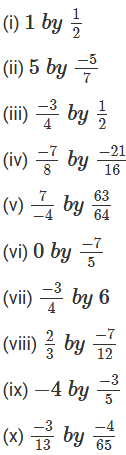Solution:Question: 2

Find the value and express as a rational number in standard form: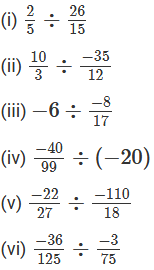Solution: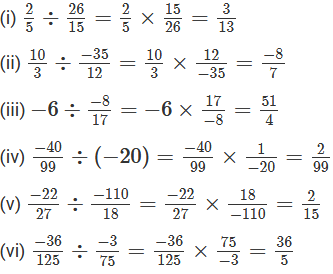Question: 3

The product of two rational numbers is 15. If one of the numbers is -10. Find the other number.

Solution:

Let, the other number be x.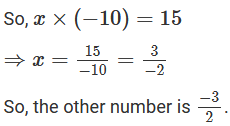Question: 4

The product of two rational numbers is −8/9. If one of the number is −4/15, Find the other number.

Solution:

Let, the other number be x.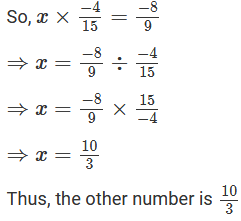Question: 5

By what number should we multiply −1/6 so that the product may be −23/9?

Solution:

Let, the number be x.Question: 6

By what number should we multiply −15/28 so that the product may be −5/7?

Solution:

Let, the number be x.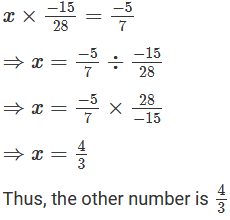Question: 7

By what number should we multiply −8/13 so that the product may be 24?

Solution:

Let, the number be x.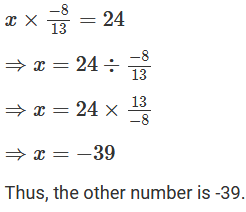Question: 8

By what number should -3/4 be multiplied in order to produce 2/3?

Solution:

Let, the number be x.Question: 9

Find (x + y) ÷ (x – y), ifSolution:Question: 10

The cost of 7(2/3) metres of rope is Rs 12(3/4). Find its cost per metres.

Solution:

The cost of 7(2/3) metres of rope is Rs. 7(2/3).

Therefore,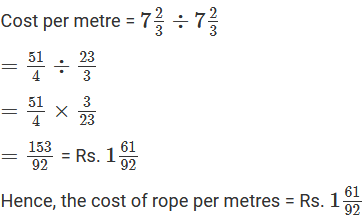Question: 11

The cost of 2(1/3) metres of cloth is Rs. 75(1/4). Find the cost of cloth per metres.

Solution:

The cost of 2(1/3) metres of cloth is Rs. 75(1/4).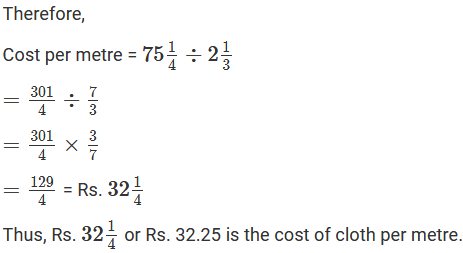Question: 12

By what number should −33/16 be divided to get -1/14?

Solution: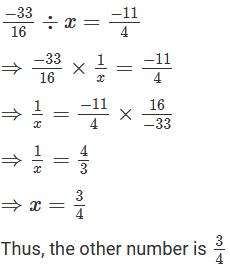Question: 13

Divide the sum of −13/5 and 12/7 by the product of −3/17 and −1/2?

Solution:Question: 14

Divide the sum of 65/12 and 12/7 by their differences.

Solution: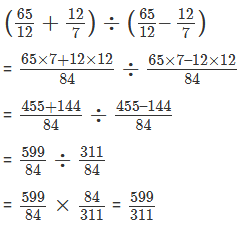Question: 15

If 24 trousers of equal size can be prepared in 54 meters of cloth, what length of cloth is required for each trouser?

Solution:

Cloth needed to prepare 24 trousers = 54 m

So,

Length of the cloth required for each trousers = 54 ÷ 24 = 54/24 = 9/4 m = 2(1/4) metres.### Course Features

• 728 Video Lectures
• Revision Notes
• Previous Year Papers
• Mind Map
• Study Planner
• NCERT Solutions
• Discussion Forum
• Test paper with Video Solution